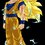# Doubt in a combinatorics problem

Suppose we have a set $S=\{2,3,4,5,6,7,8,9\}$. In how many ways, can we select 4 pairs of numbers from $S$ such that the greatest common divisor of the two numbers in each pair is not equal to 2?5 years, 3 months ago

This discussion board is a place to discuss our Daily Challenges and the math and science related to those challenges. Explanations are more than just a solution — they should explain the steps and thinking strategies that you used to obtain the solution. Comments should further the discussion of math and science.

When posting on Brilliant:

• Use the emojis to react to an explanation, whether you're congratulating a job well done , or just really confused .
• Ask specific questions about the challenge or the steps in somebody's explanation. Well-posed questions can add a lot to the discussion, but posting "I don't understand!" doesn't help anyone.
• Try to contribute something new to the discussion, whether it is an extension, generalization or other idea related to the challenge.

MarkdownAppears as
*italics* or _italics_ italics
**bold** or __bold__ bold
- bulleted- list
• bulleted
• list
1. numbered2. list
1. numbered
2. list
Note: you must add a full line of space before and after lists for them to show up correctly
paragraph 1paragraph 2

paragraph 1

paragraph 2

[example link](https://brilliant.org)example link
> This is a quote
This is a quote
    # I indented these lines
# 4 spaces, and now they show
# up as a code block.

print "hello world"
# I indented these lines
# 4 spaces, and now they show
# up as a code block.

print "hello world"
MathAppears as
Remember to wrap math in $$ ... $$ or $ ... $ to ensure proper formatting.
2 \times 3 $2 \times 3$
2^{34} $2^{34}$
a_{i-1} $a_{i-1}$
\frac{2}{3} $\frac{2}{3}$
\sqrt{2} $\sqrt{2}$
\sum_{i=1}^3 $\sum_{i=1}^3$
\sin \theta $\sin \theta$
\boxed{123} $\boxed{123}$

Sort by:

Can a number be repeated in the pairs?

- 5 years, 2 months ago

No.

- 5 years, 2 months ago

Case 1: One of the pairs is (4,8): $4\times {4\choose2}$

Still working on Case 2.

- 5 years, 2 months ago

Case 2:$4!$.

So, on the whole $\boxed{ 48 }$ ways. Am I right?

- 5 years, 2 months ago

You see,I do not know the correct answer.But,according to me the answer cannot be so less.Just to prove my point,let us assume that 2 is paired with 3.So,we have our first pair (2,3) whose gcd is not 2.Now,we can make the remaining three pairs using 4,5,6,7,8,9 ; but we must ensure that the pair (4,6) or (6,8) is not selected.So,the total number of ways of selecting the remaining three pairs is (6C2)(4C2)(2C2) - 2 (4C2)(2C2)=78.But,this is only one possible case (many other cases still remain to be considered).So, the answer must be greater than 78.Does this make sense?

- 5 years, 2 months ago

Here's mine.

Case 1: Group 2 with any number other than 6 (since 4 and 8 are alredy grouped) and group the remaining among themselves in any manner.

Case 2: None of the evens are paired. So, the pairing of the odds with the evens can be done in 4! ways.

Edit: I get your arguement. But then, what's the flaw in mine?

- 5 years, 2 months ago

You have added the number of ways of case 1 and case 2 incorrectly.Even by your method the answer is 36.

- 5 years, 2 months ago

I think in your argument you didn't take into consideration that we have to just select the pairs. Because all the 3 remaining pairs are distinct, it would not be 78, but would be 13 instead.

- 5 years, 2 months ago

No,it should be ((6C2)(4C2)(2C2)/3!) -2 ((4C2)(2C2))/2!=9.Also,only three other cases remain in which 2 can be paired with 5,7,9 apart from 3 whose case has been considered.The number of ways of selection for the last three cases is same as that of the first case, by symmetry.Hence,the total number of ways is 9 times (4) = 36.Is it now making sense? Would the answer be 864 had order of selection mattered?

- 5 years, 2 months ago

Yes 36 seems to be correct. I too was getting the same answer but my cases were a bit different than yours. I have posted the solution. If the order of selection had mattered then answer would be 36* 4!=864.

- 5 years, 2 months ago

Yes,correct.It should be 864 had the order of selection mattered.

- 5 years, 2 months ago

I was getting the answer as 36.

My cases were similar to that of Deeparaj.

Case 1: When (4,8) is one of the selected pair.

Among the remaining 6 numbers only (2,6) have GCD=2. We can select any 3 pairs from the remaining 6 numbers in ((6C2)(4C2)(2C2)/3!)=15 ways( Note that we have to only select the pairs, hence the factor of 3! in the denominator). From this we need to subtract the ways where (2,6) is one of the pairs. Hence the answer of case 1 is 15-3=12.

Case 2: When (4,8) is not of the pairs.

In this case we can show that in each of the 4 pairs we must have one odd number and one even. Therefore total number ways of selecting 4 pairs in this case is simply 4!=24.

- 5 years, 2 months ago

Ah...

I forgot to divide by 2! in my first case to remove the ordering. Thanks for the clarification.

- 5 years, 2 months ago

- 4 years, 11 months ago

- 4 years, 11 months ago